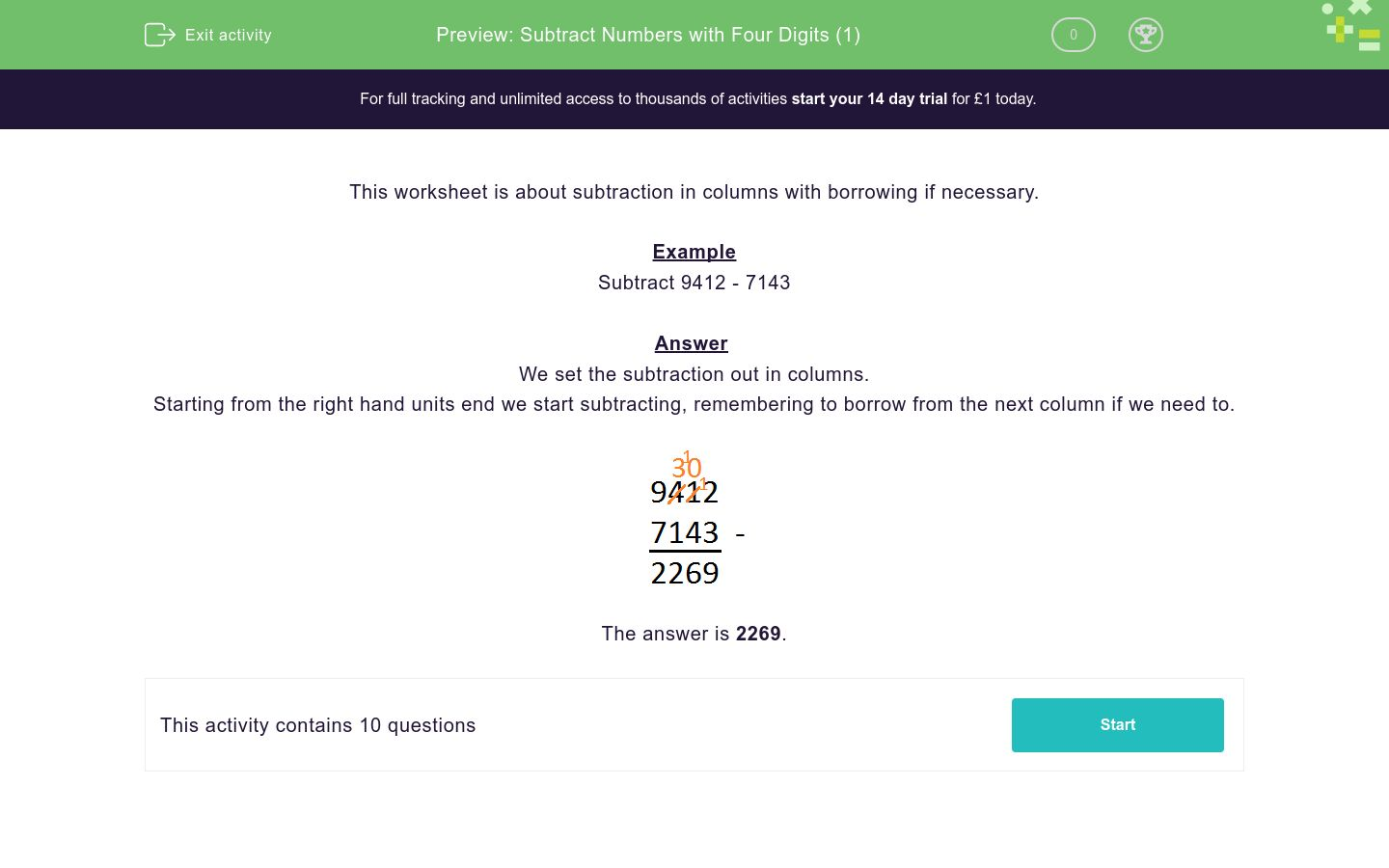# Subtract Numbers with Four Digits (1)

In this worksheet, students subtract numbers with four digits in columns with borrowing if necessary.Key stage:  KS 2

Curriculum topic:   Number: Addition and Subtraction

Curriculum subtopic:   Add/Subtract up to Four-Digit Numbers

Difficulty level:### QUESTION 1 of 10

This worksheet is about subtraction in columns with borrowing if necessary.

Example

Subtract 9412 - 7143

We set the subtraction out in columns.

Starting from the right hand units end we start subtracting, remembering to borrow from the next column if we need to.